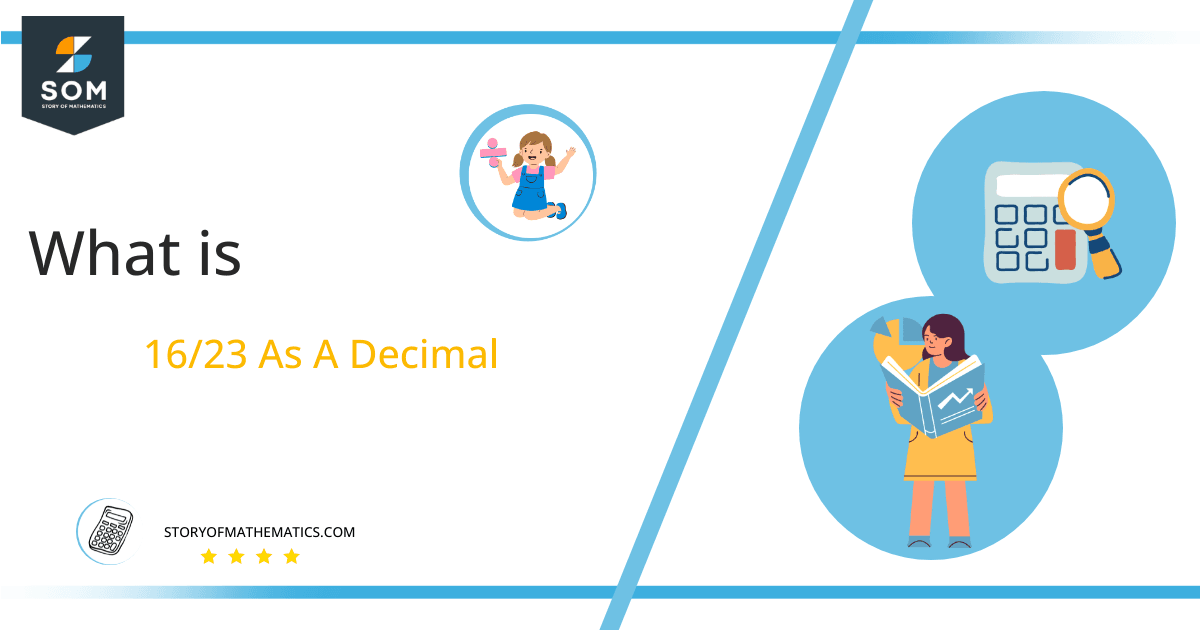# What Is 16/23 as a Decimal + Solution With Free Steps

The fraction 16/23 as a decimal is equal to 0.695.

The Fractions and decimals are two types of representation of the division’s result. The fractions are expressed as p/q, whereas decimals are expressed as a.bcd.

Here, we are more interested in the division types that result in a Decimal value, as this can be expressed as a Fraction. We see fractions as a way of showing two numbers having the operation of Division between them that result in a value that lies between two Integers.Now, we introduce the method used to solve said fraction to decimal conversion, called Long Division, which we will discuss in detail moving forward. So, let’s go through the Solution of fraction 16/23.

## Solution

First, we convert the fraction components, i.e., the numerator and the denominator, and transform them into the division constituents, i.e., the Dividend and the Divisor, respectively.

This can be done as follows:

Dividend = 16

Divisor = 23

Now, we introduce the most important quantity in our division process: the Quotient. The value represents the Solution to our division and can be expressed as having the following relationship with the Division constituents:

Quotient = Dividend $\div$ Divisor = 16 $\div$ 23

This is when we go through the Long Division solution to our problem. Given is the Long division process in figure 1:Figure 1

## 16/23 Long Division Method

We start solving a problem using the Long Division Method by first taking apart the division’s components and comparing them. As we have 16 and 23, we can see how 16 is Smaller than 23, and to solve this division, we require that 16 be Bigger than 23.

This is done by multiplying the dividend by 10 and checking whether it is bigger than the divisor or not. If so, we calculate the Multiple of the divisor closest to the dividend and subtract it from the Dividend. This produces the Remainder, which we then use as the dividend later.

Now, we begin solving for our dividend 16, which after getting multiplied by 10 becomes 160.

We take this 160 and divide it by 23; this can be done as follows:

160 $\div$ 23 $\approx$ 6

Where:

23 x 6 = 138

This will lead to the generation of a Remainder equal to 160 – 138 = 22. Now this means we have to repeat the process by Converting the 22 into 220 and solving for that:

220 $\div$ 23 $\approx$ 9

Where:

23 x 9 = 207

This, therefore, produces another Remainder which is equal to 220 – 207 = 13. Now we must solve this problem to Third Decimal Place for accuracy, so we repeat the process with dividend 130.

130 $\div$ 23 $\approx$ 5

Where:

23 x 5 = 115

Finally, we have a Quotient generated after combining the three pieces of it as 0.695, with a Remainder equal to 15.Images/mathematical drawings are created with GeoGebra.